# Using energy balances to estimate body temperatures

#### 2022-09-20

# Load TrenchR package

library(TrenchR)

This tutorial covers how to construct energy balances for applications such as estimating the body temperature of organisms. The tutorial first reviews general functions to estimate forms of heat exchange and balance the exchanges. Finally, we present functions to implement specialized energy balances for particular organisms.

# Operative environmental temperatures

The body temperatures, $$T_b$$, of organisms can depart dramatically from air temperatures due to energy exchange with the environment. Heat is gained by absorbing solar and thermal radiation and from metabolic reactions. Heat is lost through the organism’s emission of radiation and the evaporation of water. The organism exchanges heat with the surrounding air or water via convection and with the ground and other solid surfaces in contact via conduction. The balance of these heat exchanges can be estimated and is often referred to as operative environmental temperature, $$T_e$$. The $$T_e$$ indicates the steady-state temperature of an organism with given physical properties in a particular environment, omitting heat gain via metabolism and heat loss via evaporation (Bakken 1992).

The following accounting of heat gains and losses at the surface of the organism can be used to estimate $$T_b$$ (or $$T_e$$ if $$Q_{met}=0$$ and $$Q_{evap}=0$$) (Gates 2012):

$Q_{net} = Q_{abs} - Q_{emit} - Q_{conv} - Q_{cond} - Q_{met} - Q_{evap},$

where $$Q_{net}$$ is the net energy exchange with the environment (W), $$Q_{abs}$$ is the solar radiation absorbed (W), $$Q_{emit}$$ is the net thermal radiation emitted (W), $$Q_{conv}$$ is energy exchange due to convection (W), $$Q_{cond}$$ is energy exchange due to conduction (W), $$Q_{met}$$ is the energy emitted due to metabolism (W), and $$Q_{evap}$$ is the energy emitted due to evaporative water loss (W).

The energy balance is available in TrenchR as follows:

Qnet_Gates(Qabs  = 500,
Qemit = 10,
Qconv = 100,
Qcond = 100,
Qmet  = 10,
Qevap = 5)
##  275

We briefly review each component of the energy balance below. Details are available in biophysical ecology texts (Gates 2012; Campbell and Norman 2012).

The solar radiation absorbed by animals, $$Q_{abs} (W)$$, is the sum of direct $$S_{dir}$$, diffuse $$S_{dif}$$, and reflected $$S_{ref}$$ solar radiation ($$W/m^2$$). The sum is weighted by the organism’s surface area $$A (m)$$ exposed to the radiation sources. Additionally, all forms of incoming radiation are multiplied by the solar absorptivity of the animal surface ($$a$$ proportion) to estimate absorbed radiation. The summation of incoming solar radiation is thus as follows:

$Qabs= a \times A_{dir} \times S_{dir} + a \times A_{dif} \times S_{dif} + a \times A_{ref} \times S_{ref},$

where $$A_{dir}$$, $$A_{dif}$$, and $$A_{ref}$$ are the surface areas exposed to direct, diffuse, and reflected solar radiation, respectively.

The summation is available in R as follows:

plot(x    = seq(100, 1000, 100),
A       = 1,
psa_dir = 0.4,
psa_ref = 0.4,
S_dir   = seq(100, 1000, 100),
S_dif   = 200,
a_s     = 0.5),
type = "l",
xlab = expression("direct solar radiation" ~ (W/m^{2})),
ylab = "solar radiation absorbed (W)")

points(x    = seq(100, 1000, 100),
A       = 1,
psa_dir = 0.2,
psa_ref = 0.4,
S_dir   = seq(100, 1000, 100),
S_dif   = 200,
a_s     = 0.5),
type = "l",
lty  = "dashed")

points(x    = seq(100, 1000, 100),
A       = 1,
psa_dir = 0.4,
psa_ref = 0.4,
S_dir   = seq(100, 1000, 100),
S_dif   = 200,
a_s     = 0.5),
type = "l",
lty  = "dotted")

points(x    = seq(100, 1000, 100),
A = 1,
psa_dir = 0.4,
psa_ref = 0.4,
S_dir = seq(100, 1000, 100),
S_dif = 200,
a_s = 0.2),
type = "l",
lty  = "dotdash")

legend(x      = "topleft",
title  = "parameters",
legend = c("a = 0.9, psa_dir = 0.4, a_s = 0.5", "a = 0.9, sa_dir = 0.2, a_s = 0.5", "a = 0.45, psa_dir = 0.4, a_s = 0.5", "a = 0.9, psa_dir = 0.4, a_s = 0.2"),
lty    = c("solid", "dashed", "dotted", "dotdash"))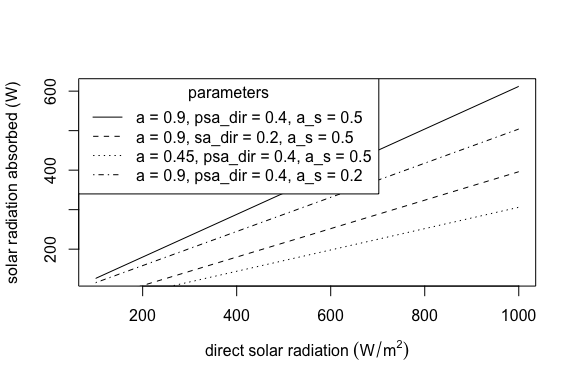In the functions, psa_dir and psa_ref are the proportions of surface area exposed to direct and reflected solar radiation, respectively. We assume psa_dif = psa_dir. The functions proportion_silhouette_area() and proportion_silhouette_area_shapes() provide assistance calculating the proportional of surface area exposed via estimating silhouette area. Additionally, see the microclimate tutorial for tools for estimating incoming solar radiation.

The net rate of emission of thermal radiation from the surface of an animal, $$Q_{emit} (W)$$, is determined by the difference between the surface temperature of the animal $$T_b (K)$$ and the temperatures of the air $$T_a (K)$$ and of the ground $$T_g (K)$$. $$T_a$$ is additionally used to estimate sky temperature $$T_{sky} (K)$$, the effective radiant temperature of the sky as $$T_{sky}= 1.22\times (T_a-273.15)-20.4+273.15$$ (Gates 2012). The following expressions can be used to estimate $$Q_{emit} (W)$$ for animals in enclosed and open environments, respectively: $enclosed: Q_{emit}= A_r \epsilon \sigma (T_b^4 - T_a^4)\\ open: Q_{emit}= \epsilon \sigma (A_s (T_b^4 - T_{sky}^4)+A_r (T_b^4 - T_g^4)),$

where $$A_s$$ and $$A_r$$ are the areas ($$m^2$$) exposed to the sky (or enclosure) and the ground, respectively; $$\epsilon$$ is the longwave infrared emissivity of skin [proportion, 0.95 to 1 for most animals (Gates 2012)]; and $$\sigma$$ is the Stefan-Boltzmann constant ($$5.673\times10^{-8} W m^{-2} K^{-4}$$).

The function is available in R as follows:

plot(x    = 293:313,
A        = 1,
psa_dir  = 0.4,
psa_ref  = 0.6,
T_b      = 293:313,
T_g      = 293,
T_a      = 298,
enclosed = FALSE),
type = "l",
xlab = "body surface temperature, Tb (K)",
ylab = "emitted thermal radiation, Qemit (W)")

points(x    = 293:313,
A        = 1,
psa_dir  = 0.2,
psa_ref  = 0.8,
T_b      = 293:313,
T_g      = 293,
T_a      = 298,
enclosed = TRUE),
type = "l",
lty  = "dashed")

points(x    = 293:313,
A        = 1,
psa_dir  = 0.4,
psa_ref  = 0.8,
T_b      = 293:313,
T_g      = 283,
T_a      = 298,
enclosed = FALSE),
type = "l",
lty  = "dotted")

points(x    = 293:313,
A = 1,
psa_dir = 0.2,
psa_ref = 0.6,
T_b      = 293:313,
T_g      = 283,
T_a      = 298,
enclosed = FALSE),
type = "l",
lty  = "dotdash")

legend(x      = "topleft",
title  = "parameters",
legend = c("psa_dir= 0.4, T_g = 293", "psa_dir= 0.2, T_g = 293", "psa_dir= 0.4, T_g = 283", "psa_dir= 0.2, T_g = 283"),
lty    = c("solid", "dashed", "dotted", "dotdash"))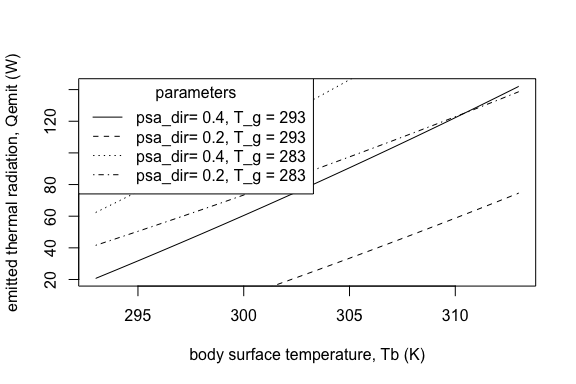# Convection, Qconv

Animals exchange heat with the air or water they are immersed in at a rate determined by the temperature difference between the temperature of the animal, $$T_b (K)$$, and that of the air or water, $$T_a (K)$$. The rate is also determined by the animal’s surface area exposed to the air or water, which we estimate from the input parameters of the animal’s surface area, $$A (m^2)$$, and the proportion of the surface area in contact with the surrounding fluid. A heat transfer coefficient, $$H_L (W m^{-2} K^{-1})$$, which can be estimated using the relations below, is used to quantify the rate of heat exchange. An enhancement factor multiplier, $$ef$$, can also be incorporated to account for increases in heat exchange resulting from air turbulence in field conditions. Conduction can be estimated as follows:

$Q_{conv}= ef\cdot H_L(A\cdot \mbox{proportion})(T_a-T_b).$

The function is available in R:

plot(x    = 293:313,
y    = Qconvection(T_a        = 303,
T_b        = 293:313,
H          = 5,
A          = 0.0025,
proportion = 0.6),
type = "l",
xlab = "ground temperature (K)",
ylab = "Q convection (W)")

points(x    = 293:313,
y    = Qconvection(T_a        = 303,
T_b        = 293:313,
H          = 15,
A          = 0.0025,
proportion = 0.6),
type = "l",
lty  = "dashed")

points(x    = 293:313,
y    = Qconvection(T_a        = 303,
T_b        = 293:313,
H          = 5,
A          = 0.0025,
proportion = 0.3),
type = "l",
lty  = "dotted")

points(x    = 293:313,
y    = Qconvection(T_a        = 303,
T_b        = 293:313,
H          = 15,
A          = 0.0025,
proportion = 0.3),
type = "l",
lty  = "dotdash")

legend(x      = "topleft",
title  = "parameters",
legend = c("H = 5, proportion = 0.6", "H = 15, proportion = 0.6", "H = 5, proportion = 0.3", "H = 15, proportion = 0.3"),
lty    = c("solid", "dashed", "dotted", "dotdash"))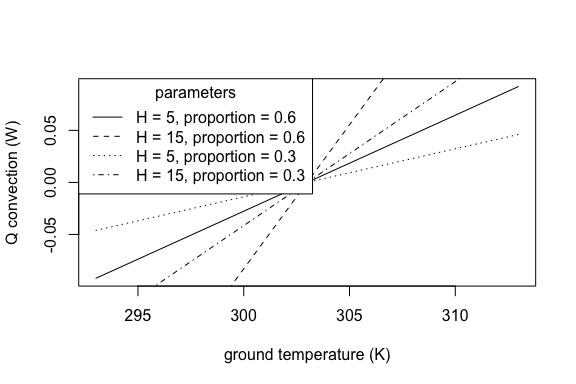The convective heat transfer coefficient depends on whether the heat exchange is free or forced (due to fluid flow). The variables involved in heat transfer can be combined into dimensionless groups that are used to compare the scales of heat exchange processes and thus whether modeling free or forced convection is more appropriate. We provide the primary dimensionless numbers.

The Nusselt number provides a dimensionless estimate of conductance and is estimated as $$Nu = H_L \cdot D / K$$, where $$H_L$$ is the convective heat transfer coefficient ($$W m^{-2} K^{-1}$$), $$D$$ is the characteristic dimension ($$m$$), and $$K$$ is thermal conductivity ($$W K^{-1}m^{-1}$$).

The Prandtl number describes the ratio of kinematic viscosity to thermal diffusivity as $$Pr= c_p \mu /K$$, where $$c_p$$ is the specific heat at constant pressure ($$J mol^{-1} K^{-1}$$) and $$\mu$$ is dynamic viscosity ($$mol \:s^{-1}m^{-1}$$).

The Reynolds Number indicates the amount of turbulence in air or water flows and is estimated as the ratio of internal viscous forces: $$Re = VD / \nu$$, where $$V$$ is wind speed ($$m/s$$), $$D$$ is the characteristic dimension ($$m$$), and $$\nu$$ is the kinematic viscosity ($$m^2 s^{-1}$$). Kinematic viscosity is the ratio of dynamic viscosity to the density of the fluid.

The Grashof number describes the ability of a parcel of fluid warmer or colder than the surrounding fluid to rise against or fall with the attractive force of gravity. It is estimated as the ratio of a buoyant force times an inertial force to the square of a viscous force: $$Gr = g D^3 \frac{\mid Tg-Ta \mid}{Ta {\nu}^2}$$, where $$g$$ is gravitational acceleration ($$g= 9.8 m/s$$).

The dimensionless groups are available in R as follows:

plot(x    = 1:30,
y    = Nusselt_number(H_L = 1:30,
D   = 0.01,
K   = 0.5),
type = "l",
xlab = expression("heat transfer coefficient" ~ (W ~ m^{-2} ~ K^{-1})),
ylab = "Nusselt number")

points(x    = 1:30,
y    = Nusselt_number(H_L = 1:30,
D   = 0.01,
K   = 1.5),
type = "l",
lty  = "dashed")

points(x    = 1:30,
y    = Nusselt_number(H_L = 1:30,
D   = 0.05,
K   = 0.5),
type = "l",
lty  = "dotted")

points(x    = 1:30,
y    = Nusselt_number(H_L = 1:30,
D   = 0.05,
K   = 1.5),
type = "l",
lty  = "dotdash")

legend(x      = "topleft",
title  = "parameters",
legend = c("D = 0.01, K = 0.5", "D = 0.01, K = 1.5", "D = 0.05, K = 0.5", "D = 0.05, K = 1.5"),
lty    = c("solid", "dashed", "dotted", "dotdash"))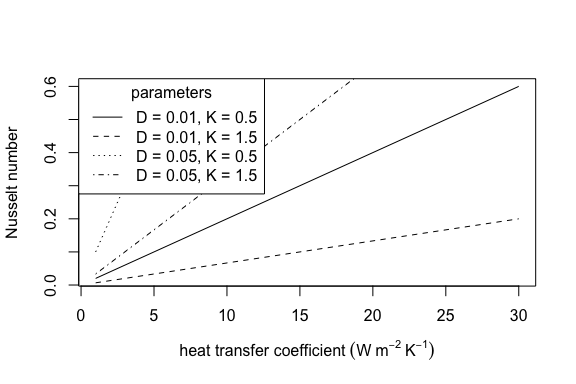plot(x    = 1:30,
y    = Prandtl_number(c_p = 1:30,
mu  = 0.00001,
K   = 0.5),
type = "l",
xlab = expression("specific heat at constant pressure" ~ (J ~ mol^{-1} ~ K^{-1})),
ylab = "Prandtl number")

points(x    = 1:30,
y    = Prandtl_number(c_p = 1:30,
mu  = 0.00002,
K   = 0.5),
type = "l",
lty  = "dashed")

points(x    = 1:30,
y    = Prandtl_number(c_p = 1:30,
mu  = 0.00001,
K   = 1.5),
type = "l",
lty  = "dotted")

points(x    = 1:30,
y    = Prandtl_number(c_p = 1:30,
mu  = 0.00002,
K   = 1.5),
type = "l",
lty  = "dotdash")

legend(x      = "topleft",
title  = "parameters",
legend = c("mu = 0.00001, K = 0.5", "mu = 0.00002, K = 0.5", "mu = 0.00001, K = 1.5", "mu = 0.00002, K = 1.5"),
lty    = c("solid", "dashed", "dotted", "dotdash"))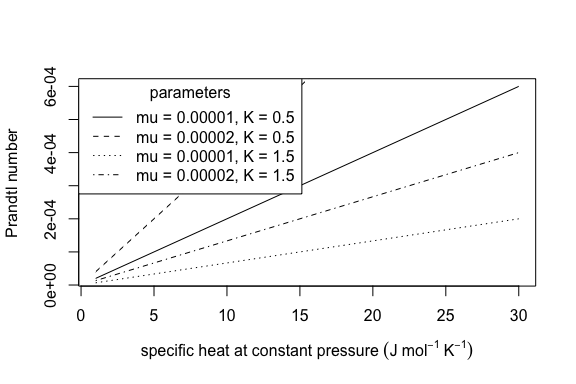plot(x    = seq(0, 5, 0.2),
y    = Reynolds_number(u  = seq(0, 5, 0.2),
D  = 0.001,
nu = 1),
type = "l",
xlab = "wind speed (m/s)",
ylab = "Reynolds number")

points(x    = seq(0, 5, 0.2),
y    = Reynolds_number(u  = seq(0, 5, 0.2),
D  = 0.001,
nu = 1.5),
type = "l",
lty  = "dashed")

points(x    = seq(0, 5, 0.2),
y    = Reynolds_number(u  = seq(0, 5, 0.2),
D  = 0.002,
nu = 1),
type = "l",
lty  = "dotted")

points(x    = seq(0, 5, 0.2),
y    = Reynolds_number(u  = seq(0, 5, 0.2),
D  = 0.002,
nu = 1.5),
type = "l",
lty  = "dotdash")

legend(x      = "topleft",
title  = "parameters",
legend = c("D = 0.001, nu = 1", "D = 0.001, nu = 1.5", "D = 0.002, nu = 1", "D = 0.002, nu = 1.5"),
lty    = c("solid", "dashed", "dotted", "dotdash"))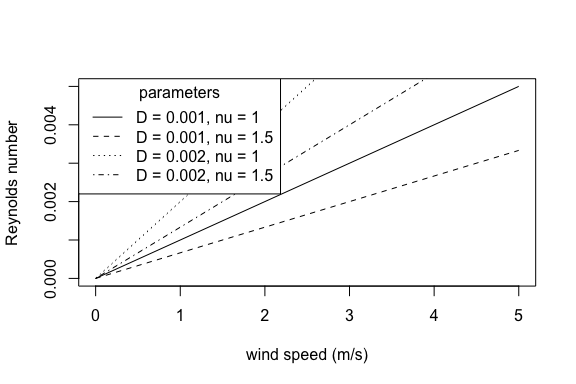plot(x    = seq(0, 0.01, 0.001),
y    = Grashof_number(T_a = 30,
T_g = 35,
D   = seq(0, 0.01, 0.001),
nu  = 1.0),
type = "l",
xlab = "characteristic dimension (m)",
ylab = "Grashof number")

points(x    = seq(0, 0.01, 0.001),
y    = Grashof_number_Gates(T_a  = 30,
T_g  = 35,
beta = 0.00367,
D    = seq(0, 0.01, 0.001),
nu   = 1.0),
type = "l",
col = "red")

points(x    = seq(0, 0.01, 0.001),
y    = Grashof_number(T_a  = 30,
T_g  = 35,
D  = seq(0, 0.01, 0.001),
nu   = 1.4),
type = "l",
lty = "dashed")

points(x    = seq(0, 0.01, 0.001),
y    = Grashof_number_Gates(T_a  = 30,
T_g  = 35,
beta = 0.00367,
D    = seq(0, 0.01, 0.001),
nu   = 1.4),
type = "l",
col = "red",
lty = "dashed")

legend(x      = "topleft",
title  = "parameters",
legend = c("Grashof_number, nu = 1", "Grashof_number_Gates, nu = 1", "Grashof_number, nu = 1.4", "Grashof_number_Gates, nu = 1.4"),
lty    = c("solid", "dashed", "dotted", "dotdash"))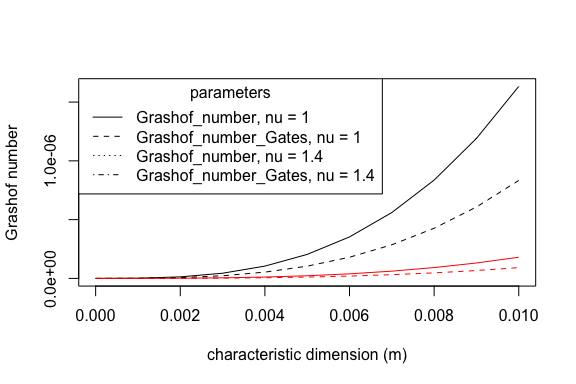Relations among the dimensionless groups can also be used for estimation. Empirically-derived relationships can be used to estimate the Nusselt number $$Nu$$ from the Reynolds number $$Re$$ or the Grashof number $$Gr$$. Comparisons of $$Gr$$ and $$Re$$ allow determining whether modeling free or forced convection is appropriate. Forced convection is appropriate when $$Gr \leq 16 Re^2$$. For convenience, we provide the relationships as R functions:

plot(x    = 1:10,
y    = Nusselt_from_Reynolds(Re    = 1:10,
taxon = "sphere"),
type = "l",
xlab = "Re",
ylab = "Nu",
ylim = c(0,4))

points(x    = 1:10,
y    = Nusselt_from_Reynolds(Re    = 1:10,
taxon = "cylinder"),
type = "l",
lty  = "dashed")

points(x    = 1:10,
y    = Nusselt_from_Reynolds(Re    = 1:10,
taxon = "frog"),
type = "l",
lty  = "dotted")

points(x    = 1:10,
y    = Nusselt_from_Reynolds(Re    = 1:10,
taxon = "lizard_traverse_to_air_flow"),
type = "l",
lty  = "dotdash")

points(x    = 1:10,
y    = Nusselt_from_Reynolds(Re    = 1:10,
taxon = "lizard_parallel_to_air_flow"),
type = "l",
lty  = "dotdash",
col = "red")

points(x    = 1:10,
y    = Nusselt_from_Reynolds(Re    = 1:10,
taxon = "lizard_surface"),
type = "l",
lty  = "dotdash",
col = "orange")

points(x    = 1:10,
y    = Nusselt_from_Reynolds(Re    = 1:10,
taxon = "lizard_elevated"),
type = "l",
lty  = "dotdash",
col = "green")

points(x    = 1:10,
y    = Nusselt_from_Reynolds(Re    = 1:10,
taxon = "flyinginsect"),
type = "l",
lty  = "longdash")

points(x    = 1:10,
y    = Nusselt_from_Reynolds(Re    = 1:10,
taxon = "spider"),
type = "l",
lty  = "twodash")

legend(x      = "topright",
title  = "taxa",
legend = c("sphere", "cylinder", "frog", "lizard_traverse_to_air_flow", "lizard_parallel_to_air_flow", "lizard_surface", "lizard_elevated", "flyinginsect", "spider"),
lty    = c("solid", "dashed", "dotted", "dotdash", "dotdash", "dotdash", "dotdash", "longdash", "twodash"),
col    = c("black", "black", "black", "black", "red", "orange", "green", "black", "black" ))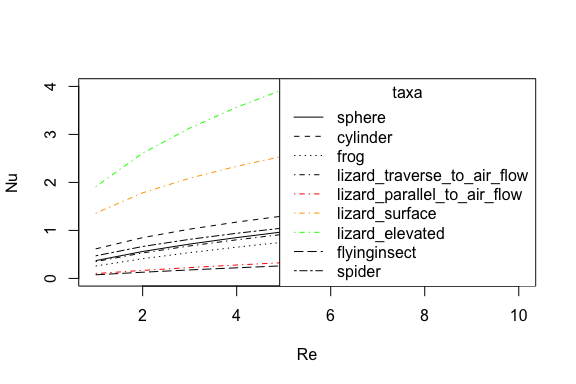Nusselt_from_Grashof(Gr = 5)
##  0.7177674
free_or_forced_convection(Gr = 100,
Re = 5)
##  "intermediate condition, mixed convection based on Nusselt numbers is appropriate"

We offer methods to estimate the convective heat transfer coefficient based on either empirical measurements (heat_transfer_coefficient()) or approximating the animal shape as a sphere (heat_transfer_coefficient_approximation()). Approximating the animal shape as a sphere enables simplification while also producing a reasonable approximation (Mitchell 1976). The functions approximate forced convective heat transfer as a function of windspeed $$V (m/s)$$, the characteristic dimension $$D (m)$$, the thermal conductivity of the air $$K (W m^{-1} K^{-1})$$, the kinematic viscosity of the air $$\nu (m^2 s^{-1})$$, and the taxa or a generic shape. An additional, simplified function (heat_transfer_coefficient_simple()) provides a reasonable approximation based on $$V$$ and $$D$$ for most environmental conditions.

oldpar <- par()

par(mar = c(5,  5, 3, 2))

plot(x    = seq(0, 3, 0.25),
y    = heat_transfer_coefficient(V     = seq(0, 3, 0.25),
D     = 0.05,
K     =  25.7 * 10^(-3),
nu    =  15.3 * 10^(-6),
taxon = "sphere"),
type = "l",
xlab = "Air velocity (m/s)",
ylab = expression("heat transfer coefficient," ~ H[L] ~ (W ~ m^{-2} ~ K^{-1})))

points(x    = seq(0, 3, 0.25),
y    = heat_transfer_coefficient(V     = seq(0, 3, 0.25),
D     = 0.05,
K     =  25.7 * 10^(-3),
nu    =  15.3 * 10^(-6),
taxon = "cylinder"),
type = "l",
lty  = "dashed")

points(x    = seq(0, 3, 0.25),
y    = heat_transfer_coefficient(V     = seq(0, 3, 0.25),
D     = 0.05,
K     =  25.7 * 10^(-3),
nu    =  15.3 * 10^(-6),
taxon = "frog"),
type = "l",
lty  = "dotted")

points(x    = seq(0, 3, 0.25),
y    = heat_transfer_coefficient(V     = seq(0, 3, 0.25),
D     = 0.05,
K     =  25.7 * 10^(-3),
nu    =  15.3 * 10^(-6),
taxon = "lizard_surface"),
type = "l",
lty  = "dotdash")

points(x    = seq(0, 3, 0.25),
y    = heat_transfer_coefficient(V     = seq(0, 3, 0.25),
D     = 0.05,
K     =  25.7 * 10^(-3),
nu    =  15.3 * 10^(-6),
taxon = "lizard_elevated"),
type = "l",
lty  = "dotdash")

points(x    = seq(0, 3, 0.25),
y    = heat_transfer_coefficient(V     = seq(0, 3, 0.25),
D     = 0.05,
K     =  25.7 * 10^(-3),
nu    =  15.3 * 10^(-6),
taxon = "flyinginsect"),
type = "l",
lty  = "longdash")

points(x    = seq(0, 3, 0.25),
y    = heat_transfer_coefficient(V     = seq(0, 3, 0.25),
D     = 0.05,
K     =  25.7 * 10^(-3),
nu    =  15.3 * 10^(-6),
taxon = "spider"),
type = "l",
lty  = "twodash")

legend(x      = "bottomright",
title  = "taxa",
legend = c("sphere", "cylinder", "frog", "lizard_surface", "lizard_elevated", "flyinginsect", "spider"),
lty    = c("solid", "dashed", "dotted", "dotdash", "dotdash", "dotdash", "dotdash", "longdash", "twodash"))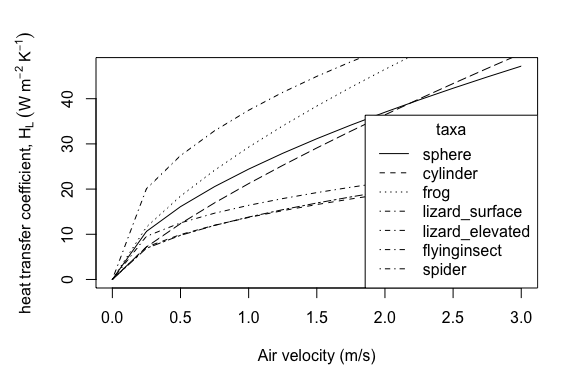par(mar = c(5,  5, 3, 2))

plot(x    = seq(0, 3, 0.25),
y    = heat_transfer_coefficient_approximation(V     = seq(0, 3, 0.25),
D     = 0.05,
K     =  25.7 * 10^(-3),
nu    =  15.3 * 10^(-6),
taxon = "sphere"),
type = "l",
xlab = "Air velocity (m/s)",
ylab = expression("heat transfer coefficient," ~ H[L] ~ (W ~ m^{-2} ~ K^{-1})))

points(x    = seq(0, 3, 0.25),
y    = heat_transfer_coefficient_approximation(V     = seq(0, 3, 0.25),
D     = 0.05,
K     =  25.7 * 10^(-3),
nu    =  15.3 * 10^(-6),
taxon = "frog"),
type = "l",
lty  = "dashed")

points(x    = seq(0, 3, 0.25),
y    = heat_transfer_coefficient_approximation(V     = seq(0, 3, 0.25),
D     = 0.05,
K     =  25.7 * 10^(-3),
nu    =  15.3 * 10^(-6),
taxon = "lizard"),
type = "l",
lty  = "dotted")

points(x    = seq(0, 3, 0.25),
y    = heat_transfer_coefficient_approximation(V     = seq(0, 3, 0.25),
D     = 0.05,
K     =  25.7 * 10^(-3),
nu    =  15.3 * 10^(-6),
taxon = "flyinginsect"),
type = "l",
lty  = "dotdash")

points(x    = seq(0, 3, 0.25),
y    = heat_transfer_coefficient_approximation(V     = seq(0, 3, 0.25),
D     = 0.05,
K     =  25.7 * 10^(-3),
nu    =  15.3 * 10^(-6),
taxon = "spider"),
type = "l",
lty  = "longdash")

legend(x      = "bottomright",
title  = "taxa",
legend = c("sphere", "frog", "lizard", "flyinginsect", "spider"),
lty    = c("solid", "dashed", "dotted", "dotdash", "longdash"))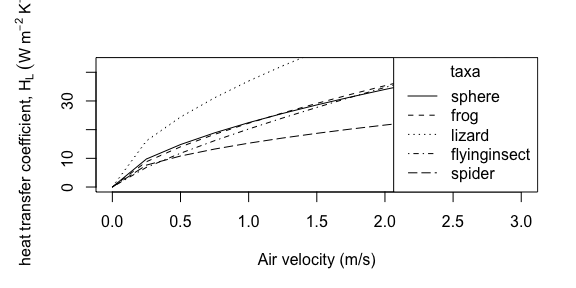plot(x    = seq(0, 3, 0.25),
y    = heat_transfer_coefficient_simple(V    = seq(0, 3, 0.25),
D    = 0.05,
type = "Spotila"),
type = "l",
xlab = "Air velocity (m/s)",
ylab = expression("heat transfer coefficient," ~ H[L] ~ (W ~ m^{-2} ~ K^{-1})))

points(x    = seq(0, 3, 0.25),
y    = heat_transfer_coefficient_simple(V    = seq(0, 3, 0.25),
D    = 0.03,
type = "Spotila"),
type = "l",
lty  = "dashed")

points(x    = seq(0, 3, 0.25),
y    = heat_transfer_coefficient_simple(V    = seq(0, 3, 0.25),
D    = 0.07,
type = "Spotila"),
type = "l",
lty  = "dotted")

legend(x      = "bottomright",
title  = "characteristic dimension (m)",
legend = c(0.03, 0.05, 0.07),
lty    = c("dashed", "solid", "dotted"))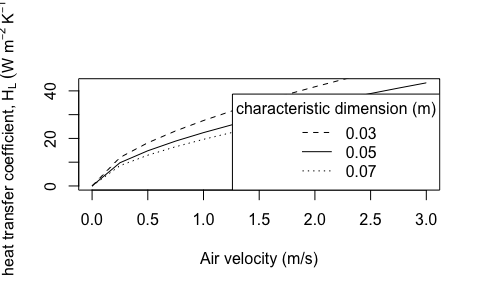# Conduction, Qcond

Animals exchange heat with the substrate and other surfaces via contact proportional to the difference between the surface temperature of the animal, $$T_b (K)$$, and the substrate temperature, $$T_s (K)$$. This conduction occurs via diffusion of heat. The extent of energy exchange is additionally determined by the area of contact and the thickness of the skin or other animal surface (assumes a well-mixed interior, see NicheMapR for approaches without this assumption). Finally, the rate of diffusion depends on the thermal conductivity of the materials in contact. Our two basic functions for estimating conduction differ in whether the rate-limiting step is the thermal conductivity of the animal [conductance_animal()] or the substrate [conductance_substrate()]. The thermal conductivity of the ground is generally greater than that of animal tissues, so animal thermal conductivity is generally the rate-limiting step and most applications should use conductance_animal().

Both functions take as parameters the animal’s surface area, $$A (m^2)$$, and the proportion of the surface area in contact with the substrate, $$\mbox{proportion}$$. When animal conductance is the rate limiting step, $$Q_{cond}$$ can be estimated as follows:

$Q_{cond}= A\cdot \mbox{proportion} \cdot K(T_b-T_g)/d,$

where $$K$$ is thermal conductivity ($$W K^{-1} m^{-1}$$), $$T_g$$ is ground (surface) temperature $$(K)$$, $$T_b$$ is body temperature $$(K)$$, and $$d$$ is the mean thickness of the animal skin (surface, $$m$$). This formulation assumes the organism has a well-mixed interior rather than an interior temperature gradient.

When substrate thermal conductivity is the rate-limiting step, $$Q_{cond}$$ can be estimated as follows:

$Q_{cond} = A\cdot \mbox{proportion} \cdot (2K_g/D)(T_b-T_g),$

where $$K_g$$ is the thermal conductivity of substrate ($$W K^{-1} m^{-1}$$) and $$D$$ is the characteristic dimension of the animal ($$m$$). The functions are available in R:

plot(x    = 293:313,
y    = Qconduction_animal(T_g        = 293:313,
T_b        = 303,
d          = 10^-6,
K          = 0.3,
A          = 10^-3,
proportion = 0.2),
type = "l",
xlab = "ground temperature (K)", ylab = "Q conductance (W)")

points(x    = 293:313,
y    = Qconduction_animal(T_g        = 293:313,
T_b        = 303,
d          = 10^-6,
K          = 0.5,
A          = 10^-3,
proportion = 0.2),
type = "l",
lty  = "dashed")

points(x    = 293:313,
y    = Qconduction_animal(T_g        = 293:313,
T_b        = 303,
d          = 10^-6,
K          = 0.3,
A          = 10^-3,
proportion = 0.4),
type = "l",
lty  = "dotted")

points(x    = 293:313,
y    = Qconduction_animal(T_g        = 293:313,
T_b        = 303,
d          = 10^-6,
K          = 0.5,
A          = 10^-3,
proportion = 0.4),
type = "l",
lty  = "dotdash")

legend(x      = "topright",
title  = "parameters",
legend = c("K = 0.3, proportion = 0.2", "K = 0.5, proportion = 0.2", "K = 0.3, proportion = 0.4", "K = 0.5, proportion = 0.4"),
lty    = c("solid", "dashed", "dotted", "dotdash"))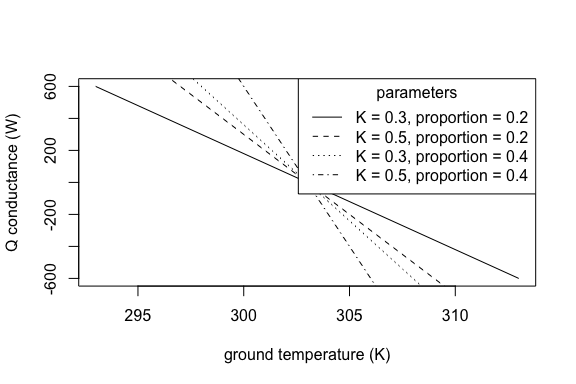plot(x    = 293:313,
y    = Qconduction_substrate(T_g        = 293:313,
T_b        = 303,
D          = 0.01,
K_g        = 0.3,
A          = 10^-2,
proportion = 0.2),
type = "l",
xlab = "ground temperature (K)",
ylab = "Q conductance (W)")

points(x    = 293:313,
y    = Qconduction_substrate(T_g        = 293:313,
T_b        = 303,
D          = 0.01,
K_g        = 0.5,
A          = 10^-2,
proportion = 0.2),
type = "l",
lty  = "dashed")

points(x    = 293:313,
y    = Qconduction_substrate(T_g        = 293:313,
T_b        = 303,
D          = 0.01,
K_g        = 0.3,
A          = 10^-2,
proportion = 0.4),
type = "l",
lty  = "dotted")

points(x    = 293:313,
y    = Qconduction_substrate(T_g        = 293:313,
T_b        = 303,
D          = 0.01,
K_g        = 0.5,
A          = 10^-2,
proportion = 0.4),
type = "l",
lty  = "dotdash")

legend(x      = "topright",
title  = "parameters",
legend = c("K = 0.3, proportion = 0.2", "K = 0.5, proportion = 0.2", "K = 0.3, proportion = 0.4", "K = 0.5, proportion = 0.4"),
lty    = c("solid", "dashed", "dotted", "dotdash"))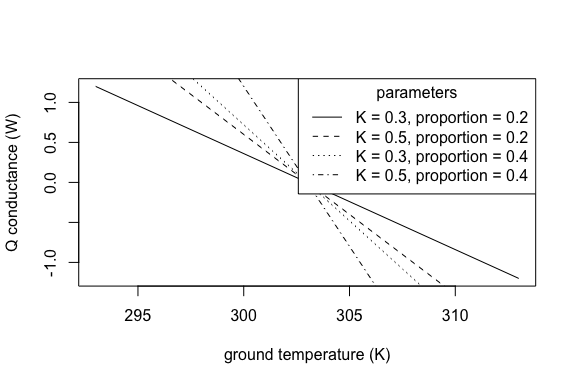# Metabolism, Qmet

Rates of heat production associated with metabolism are generally estimated using scaling relationships based on generalizing empirical data. Metabolic rates scale as a power law function of mass with an exponent less than 1 such that the per-mass metabolic rate declines with increasing mass. Metabolic rates also increase exponentially with temperature. The general form of the relationship is $$B=M^x \exp(-E_i/{kT})$$, where $$M$$ is mass, $$x$$ is an exponent approximating 0.75, $$E_i$$ is the average activation energy for the rate-limiting enzyme-catalyzed biochemical reactions of metabolism, $$k$$ is the Boltzmann constant, and $$T$$ is body temperature (Gillooly et al. 2001). We offer functions that both do not [Qmetabolism_from_mass()] and do [Qmetabolism_from_mass_temp()] include temperature dependence for a variety of taxa.

plot(x    = 1:100,
y    = Qmetabolism_from_mass(m     = 1:100,
taxon = "bird"),
type = "l",
xlab = "mass (g)",
ylab = "metabolism (W)",
log  = "xy",
ylim = c(0.1, 2))

points(x    = 1:100,
y    = Qmetabolism_from_mass(m     = 1:100,
taxon = "mammal"),
type = "l",
lty  = "dashed")

points(x    = 1:100,
y    = Qmetabolism_from_mass(m     = 1:100,
taxon = "reptile"),
type = "l",
lty  = "dotted")

legend(x      = "topleft",
title  = "taxa",
legend = c("bird", "mammal", "reptile"),
lty    = c("solid", "dashed", "dotted"))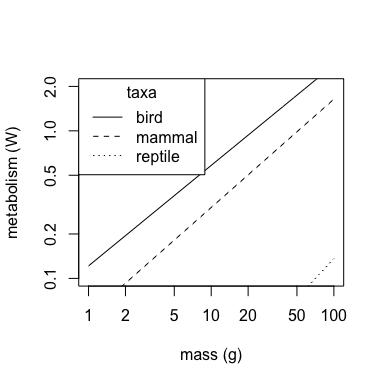plot(x    = 1:100,
y    = Qmetabolism_from_mass_temp(m     = 1:100,
T_b   = 303,
taxon = "bird"),
type = "l",
xlab = "mass (g)",
ylab = "metabolism (W)",
ylim = c(0, 0.1))

points(x    = 1:100,
y    = Qmetabolism_from_mass_temp(m     = 1:100,
T_b   = 303,
taxon = "reptile"),
type = "l",
lty  = "dotted")

points(x    = 1:100,
y    = Qmetabolism_from_mass_temp(m     = 1:100,
T_b   = 303,
taxon = "amphibian"),
type = "l",
lty  = "dotdash")

points(x    = 1:100,
y    = Qmetabolism_from_mass_temp(m     = 1:100,
T_b   = 303,
taxon = "invertebrate"),
type = "l",
lty  = "twodash")

legend(x      = "top",
title  = "taxa",
legend = c("bird,mammal", "reptile", "amphibian", "invertebrate"),
lty    = c("solid", "dotted", "dotdash", "twodash"))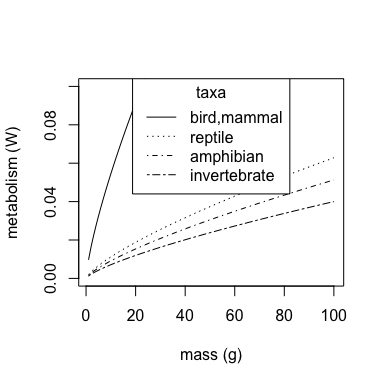plot(x    = 293:313,
y    = Qmetabolism_from_mass_temp(m     = 5,
T_b   = 293:313,
taxon = "bird"),
type = "l",
xlab = "temperature (K)",
ylab = "metabolism (W)",
ylim = c(0,0.1))

points(x    = 293:313,
y    = Qmetabolism_from_mass_temp(m     = 5,
T_b   = 293:313,
taxon = "reptile"),
type = "l",
lty  = "dotted")

points(x    = 293:313,
y    = Qmetabolism_from_mass_temp(m     = 5,
T_b   = 293:313,
taxon = "amphibian"),
type = "l",
lty  = "dotdash")

points(x    = 293:313,
y    = Qmetabolism_from_mass_temp(m     = 5,
T_b   = 293:313,
taxon = "invertebrate"),
type = "l",
lty  = "twodash")

legend(x      = "topleft",
title  = "taxa",
legend = c("bird,mammal", "reptile", "amphibian", "invertebrate"),
lty    = c("solid", "dotted", "dotdash", "twodash"))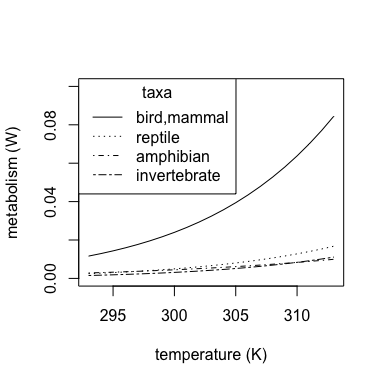# Evaporation, Qevap

We provide functions to estimate heat loss associated with evaporative water loss. We offer an empirically-derived relationship for a lizard (Porter et al. 1973; in Gates 2012) and a function based on resistances for amphibians (Spotila, O’Connor, and Bakken 1992). The function for amphibians is based on the latent heat of vaporization of water: evaporation of water results in heat loss at a rate of $$2.44 \times 10^6 J kg^{-1}$$ at biological temperatures. The rate of water loss for an amphibian with fully wetted skin can be expressed as

$E_c= A (\rho_s-h \rho_a)/r_e,$

where $$A$$ is surface area ($$m^2$$), $$r_e$$ is the external (convective) resistance to water vapor transport ($$s/m$$), $$\rho_s$$ is the saturation water vapor density at skin surface ($$kg/m^3$$), $$h$$ is relative humidity (between 0 and 1), and $$\rho_a$$ is the saturation water vapor density in ambient air ($$kg/m^3$$).

The rate of water loss for an amphibian without fully wetted skin can be expressed as $E_c= A (\rho_s-h\rho_a)/(r_i+r_e),$ where $$r_i$$ is the internal (cutaneous) resistance to vapor transport ($$s/m$$). We provide R functions for heat loss through evaporation as the product of $$E_c$$ and the latent heat of vaporization of water:

# kPa

vp <- saturation_vapor_pressure(293:313)

# convert to kg/m^3

rho_s <- vp * 0.032

# kPa

vp <- saturation_vapor_pressure(293:313-10)

# convert to kg/m^3

rho_a <-  vp * 0.032

temps      <- 293:313
Qevaps     <- rep(NA,21)
Qevaps_wet <- rep(NA,21)

for (ind in 1:21) {
Qevaps[ind]     <- Qevaporation(A     = 0.1,
T_b   = temps[ind],
taxon = "amphibian",
rho_s = rho_s[ind],
rho_a = rho_a[ind],
h     = 0.5,
H     = 20,
r_i   = 50)

Qevaps_wet[ind] <- Qevaporation(A     = 0.1,
T_b   = temps[ind],
taxon = "amphibian_wetskin",
rho_s = rho_s[ind],
rho_a = rho_a[ind],
h     = 0.5,
H     = 20,
r_i   = 50)
}

plot(x    = temps,
y    = Qevaps,
type = "l",
xlab = "body temperature (K)",
ylab = "evaporative heat loss (W)",
lty  = "dashed",
ylim = c(0, 400))

points(x    = temps,
y    = Qevaps_wet,
type = "l",
lty  = "dotted")

Qevap <- unlist(lapply(293:313,
FUN  = Qevaporation,
A = 0.1,
taxon = "lizard"))

points(x    = 293:313,
y    = Qevap,
type = "l" )

legend(x      = "right",
title  = "taxa",
legend = c("amphibian", "amphibian_wetskin", "lizard"),
lty    = c("dashed", "dotted", "solid"))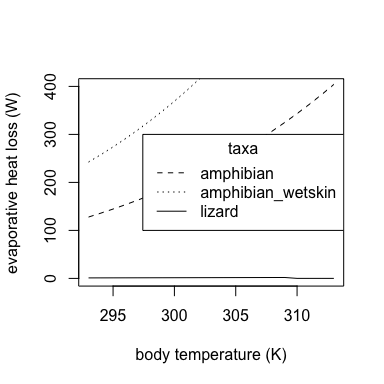We estimate external resistance to water vapor transport, $$r_e$$, using the Lewis rule describing the relationships among the coefficients for heat and mass transport: $$r_e= 0.93 \rho c_p/H_L$$, where $$\rho$$ is the density of air ($$kg m^{-3}$$), $$c_p$$ is the specific heat of air at constant pressure ($$J kg^{-1}°C^{-1}$$), and $$H_L$$ is the convective heat transfer coefficient ($$W m^{-2} °C^{-1}$$). We approximate $$\rho c_p$$as 1,200 $$J m^{-3}°C^{-1}$$ as commonly done in biological applications (Spotila, O’Connor, and Bakken 1992). We provide the relationship for $$r_e$$ as a function:

par(mar = c(5, 5, 3, 2))

plot(x    = 10:30,
y    = external_resistance_to_water_vapor_transfer(H = 10:30),
type = "l",
xlab = expression("heat transfer coefficient" ~ (W ~ m^{-2} ~ "°" ~ C^{-1})),
ylab = expression("external resistance, " ~ r[e] ~ (sm^{-1})))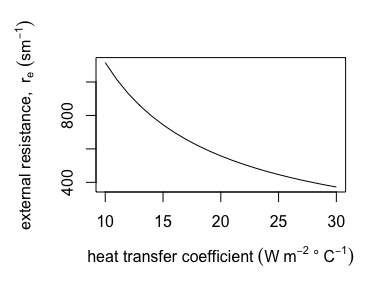At constant temperatures, the relationship between vapor pressure and vapor density is linear and vapor pressure can be approximated at temperatures between $$0°C$$ and $$40°C$$ as $$e_s= 10^{0.02604 T_a+2.82488}$$, where $$T_a$$ is air temperature ($$°C$$). We provide the approximation as a function:

plot(x    = 10:30,
y    = saturation_water_vapor_pressure(T_a = 10:30),
type = "l",
xlab = "temperature (°C)",
ylab = "saturation water vapor pressure (Pa)")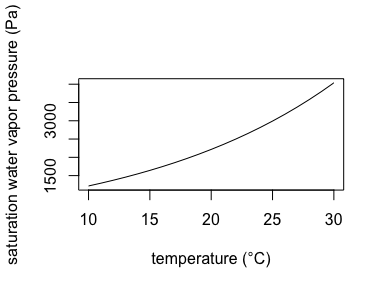# Estimating body temperatures, Tb

TrenchR primarily includes models for estimating the body temperatures (generally the operative temperatures, $$T_e$$) of organisms that have reached an equilibrium with their environment (“steady-state,” no heat retention). The following function uses the relationships above to solve the energy balance for $$Tb (K)$$.

t.seq <- lapply(293:313,
FUN     = Tb_Gates,
A       = 0.1,
D       = 0.001,
psa_dir = 0.6,
psa_ref = 0.4,
psa_air = 0.6,
psa_g   = 0.0,
T_g     = 303,
Qabs    = 10,
epsilon = 0.95,
H_L     = 10,
ef      = 1.3,
K       = 0.5)

plot(x    = 293:313,
y    = t.seq,
type = "l",
xlab = "ambient temperature, Ta (K)",
ylab = "body temperature, Tb (K)",
xlim = c(295, 315),
ylim = c(295, 315))

abline(a   = 0,
b   = 1,
col = "gray")

t.seq <- lapply(293:313,
FUN     = Tb_Gates,
A       = 0.1,
D       = 0.001,
psa_dir = 0.6,
psa_ref = 0.4,
psa_air = 0.6,
psa_g   = 0.0,
T_g     = 303,
Qabs    = 0,
epsilon = 0.95,
H_L     = 10,
ef      = 1.3,
K       = 0.5)

points(x    = 293:313,
y    = t.seq,
type = "l",
lty  = "dashed")

t.seq <- lapply(293:313,
FUN     = Tb_Gates,
A       = 0.1,
D       = 0.001,
psa_dir = 0.6,
psa_ref = 0.4,
psa_air = 0.6,
psa_g   = 0.0,
T_g     = 303,
Qabs    = 20,
epsilon = 0.95,
H_L     = 10,
ef      = 1.3,
K       = 0.5)

points(x    = 293:313,
y    = t.seq,
type = "l",
lty  = "dotted")

legend(x      = "bottomright",
legend = c(0, 10, 20),
lty    = c("dashed", "solid", "dotted"))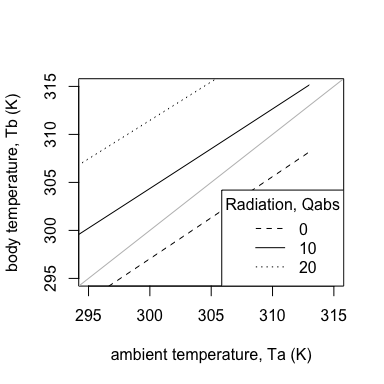Campbell and Norman (2012) use a somewhat simplified energy balance to express $$Tb$$ as a function of $$Ta$$ plus or minus a temperature increment determined by absorbed radiation, wind speed, and animal morphology:

$T_b=T_a+(S_{abs}-Q_{emit})/(c_p(g_r+g_{Ha})),$

where $$Ta$$ is air temperature $$(K)$$, $$S_{abs}$$ is the solar and thermal radiation absorbed ($$W m^{-2}$$), $$Qemit$$ is emitted thermal radiation ($$W m^{-2}$$), $$c_p$$ is the specific heat of air ($$J mol^{-1} K^{-1}$$), $$g_{r}$$ is radiative conductance $$(mol\:m^{-2} s^{-1})$$, and $$g_{Ha}$$ is the boundary conductance $$(mol\:m^{-2} s^{-1})$$. The model is based on estimating $$T_b$$ for a blackbody (perfectly absorbing) cavity with air temperatures equal to surface temperatures ($$T_a = T_s$$). The model assumes that the cavity provides the same heat load as the natural environment and thus equates metabolic heat production and evaporative cooling in the two environments. The formulation assumes a well-mixed interior of the animal and also omits conduction with the substrate. In this scenario, organisms emit thermal radiation from their surface proportional to the fourth power of $$T_a$$:

$Q_{emit}= \epsilon \sigma T_a^4 ,$

where $$\epsilon$$ is the proportional longwave infrared emissivity of skin [0.95-1 for most animals, (Gates 2012)] and $$\sigma$$ is the Stefan-Boltzmann constant ($$5.673*10^{-8} W m^{-2} K^{-4}$$).

The thermal radiation exchanged between the animals and the walls of the cavity is proportional to the temperature differences and the radiative conductance. Campbell and Norman (2012) thus use a denominator term that combines thermal radiative exchange with convective heat exchange. The radiative conductance describing the heat exchange between the core of the animal and the environment is estimated as $$g_r= 4 \sigma Ta^3/c_p(mol\:m^{-2} s^{-1})$$. The boundary conductance (heat exchange with the air via convection) is estimated assuming forced convection driven by naturally turbulent wind and an empirical approximation of animal shapes (Mitchell 1976):

$g_{Ha}(mol \:m^{-2} s^{-1})= 1.4 \times 0.135 \sqrt{(V/D)},$

where 1.4 is a factor to account for increased convection in natural environments, $$V$$ is wind speed ($$m/s$$), and $$D$$ is the characteristic dimension of the animal ($$m$$).

The function to estimate $$T_b$$ (also $$T_e$$) is available in R as follows (including the above calculations of $$Q_{emit}$$, $$g_r$$, and $$g_{Ha}$$):

plot(x    = 293:313,
y    = Tb_CampbellNorman(T_a     = 293:313,
T_g     = 303,
S       = 600,
alpha_S = 0.7,
alpha_L = 0.96,
epsilon = 0.96,
c_p     = 29.3,
D       = 0.17,
V       = 1),
type = "l",
xlab = "air temperature (K)",
ylab = "body temperature (K)",
xlim = c(295,310),
ylim = c(290,340))

abline(a   = 0,
b   = 1,
col = "gray")

points(x    = 293:313,
y    = Tb_CampbellNorman(T_a     = 293:313,
T_g     = 303,
S       = 200,
alpha_S = 0.7,
alpha_L = 0.96,
epsilon = 0.96,
c_p     = 29.3,
D       = 0.17,
V       = 1),
type = "l",
lty  = "dashed")

points(x    = 293:313,
y    = Tb_CampbellNorman(T_a     = 293:313,
T_g     = 303,
S       = 400,
alpha_S = 0.7,
alpha_L = 0.96,
epsilon = 0.96,
c_p     = 29.3,
D       = 0.17,
V       = 1),
type = "l",
lty  = "dotted")

legend(x      = "bottomright",
title  =  expression("Radiation, Sabs" ~ (W ~ m^{-2})),
legend = c(200,400,600),